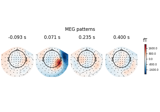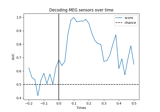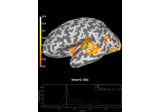# mne.decoding.LinearModel#

class mne.decoding.LinearModel(model=None)[source]#

Compute and store patterns from linear models.

The linear model coefficients (filters) are used to extract discriminant neural sources from the measured data. This class computes the corresponding patterns of these linear filters to make them more interpretable .

Parameters:
modelobject | `None`

A linear model from scikit-learn with a fit method that updates a `coef_` attribute. If None the model will be LogisticRegression.

Notes

New in version 0.10.

References

Attributes:
filters_`ndarray`, shape ([n_targets], n_features)

If fit, the filters used to decompose the data.

patterns_`ndarray`, shape ([n_targets], n_features)

If fit, the patterns used to restore M/EEG signals.

Methods

 Compute distance from the decision function of y from X. `fit`(X, y, **fit_params) Estimate the coefficients of the linear model. `fit_transform`(X, y) Fit the data and transform it using the linear model. `get_params`([deep]) Get parameters for this estimator. Compute predictions of y from X. Compute probabilistic predictions of y from X. `score`(X, y) Score the linear model computed on the given test data. `set_params`(**params) Set the parameters of this estimator. Transform the data using the linear model.
property classes_#

The classes (pass-through to model).

decision_function(X)[source]#

Compute distance from the decision function of y from X.

Parameters:
X`array`, shape (n_samples, n_features)

The data used to compute the predictions.

Returns:
y_pred`array`, shape (n_samples, n_classes)

The distances.

fit(X, y, **fit_params)[source]#

Estimate the coefficients of the linear model.

Save the coefficients in the attribute `filters_` and computes the attribute `patterns_`.

Parameters:
X`array`, shape (n_samples, n_features)

The training input samples to estimate the linear coefficients.

y`array`, shape (n_samples, [n_targets])

The target values.

**fit_params`dict` of `str` -> object

Parameters to pass to the fit method of the estimator.

Returns:
selfinstance of `LinearModel`

Returns the modified instance.

Examples using `fit`:Linear classifier on sensor data with plot patterns and filters

Linear classifier on sensor data with plot patterns and filters
fit_transform(X, y)[source]#

Fit the data and transform it using the linear model.

Parameters:
X`array`, shape (n_samples, n_features)

The training input samples to estimate the linear coefficients.

y`array`, shape (n_samples,)

The target values.

Returns:
y_pred`array`, shape (n_samples,)

The predicted targets.

get_params(deep=True)[source]#

Get parameters for this estimator.

Parameters:
deep`bool`, optional

If True, will return the parameters for this estimator and contained subobjects that are estimators.

Returns:
params`dict`

Parameter names mapped to their values.

predict(X)[source]#

Compute predictions of y from X.

Parameters:
X`array`, shape (n_samples, n_features)

The data used to compute the predictions.

Returns:
y_pred`array`, shape (n_samples,)

The predictions.

predict_proba(X)[source]#

Compute probabilistic predictions of y from X.

Parameters:
X`array`, shape (n_samples, n_features)

The data used to compute the predictions.

Returns:
y_pred`array`, shape (n_samples, n_classes)

The probabilities.

score(X, y)[source]#

Score the linear model computed on the given test data.

Parameters:
X`array`, shape (n_samples, n_features)

The data to transform.

y`array`, shape (n_samples,)

The target values.

Returns:
score`float`

Score of the linear model.

set_params(**params)[source]#

Set the parameters of this estimator.

The method works on simple estimators as well as on nested objects (such as pipelines). The latter have parameters of the form `<component>__<parameter>` so that it’s possible to update each component of a nested object.

Parameters:
**params`dict`

Parameters.

Returns:
instinstance

The object.

transform(X)[source]#

Transform the data using the linear model.

Parameters:
X`array`, shape (n_samples, n_features)

The data to transform.

Returns:
y_pred`array`, shape (n_samples,)

The predicted targets.

## Examples using `mne.decoding.LinearModel`#Decoding (MVPA)

Decoding (MVPA)Decoding source space data

Decoding source space dataLinear classifier on sensor data with plot patterns and filters

Linear classifier on sensor data with plot patterns and filters